Courses

# Determinants - Introduction JEE Notes | EduRev

## JEE : Determinants - Introduction JEE Notes | EduRev

The document Determinants - Introduction JEE Notes | EduRev is a part of JEE category.
All you need of JEE at this link: JEE

Definition

To every square matrix A = [aij] of order n, we can associate a number (real or complex) called the determinant of the matrix A, written as det A, where aij is the (i, j)th element of A.

If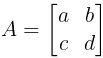, then the determinant of A, denoted by |A| (or det A), is given by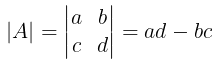Note:
(i) Only square matrices have determinants.
(ii) For a matrix A, A is read as the determinant of A and not, as a modulus of A.

1. Determinant of a matrix of order one:
Let A = [a] be the matrix of order 1.
⇒ Determinant of A = a

2. Determinant of a matrix of order two:
Let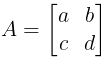be the matrix of order 2.
⇒ Determinant of A = ad-bc

3. Determinant of a matrix of order three:

• The determinant of a matrix of order three can be determined by expressing it in terms of second-order determinants which are known as the expansion of a determinant along a row (or a column).
• Consider the determinant of a square matrix A = [aij] 3×3,
i.e.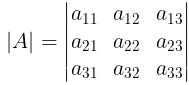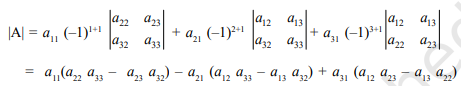Note:  In general, if A = kB, where A and B are square matrices of order n,
then |A| = kn|B|, where n = 1, 2, 3.

• We can expand the determinant through any row or column.
i.e. Δ = a11A11 + a12A12 + a13A13 = a11A11 + a21A21 + a31A31
where Aij is cofactor of aij.

Properties of Determinants

• If rows are changed into columns and columns into rows, then the values of the determinant remain unaltered.
• If any two-row (or columns) of a determinant are interchanged, the resulting determinant is the negative of the original determinant.
• If two rows (or two columns) in a determinant have corresponding elements that are equal, the value of the determinant is equal to zero.
• If each element in a row (or column) of a determinant is written as the sum of two or more terms then the determinant can be written as the sum of two or more determinants.
• If to each element of a line (row or column) of a determinant be added the equimultiples of the corresponding elements of one or more parallel lines, the determinant remains unaltered.
i.e.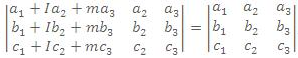• If each element in any row (or any column) of the determinant is zero, then the value of the determinant is equal to zero.
• If a determinant D vanishes for x = a, then (x - a) is a factor of D, In other words, if two rows (or two columns) become identical for x = a, then (x-a) is a factor of D.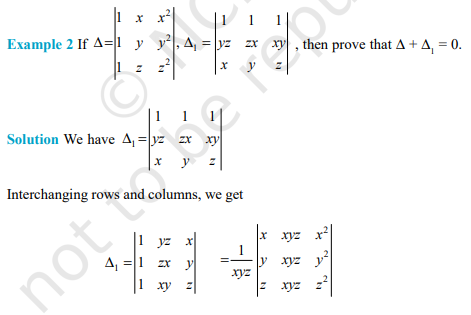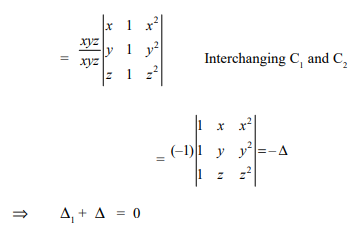Area of triangle

Assume a triangle with vertices, (x1,y1), (x2,y2), (x3,y3) in a cartisean co-ordinate system as shown below.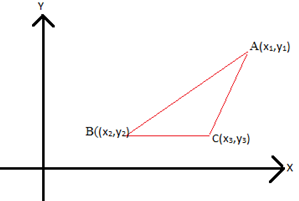Then the area of the given triangle,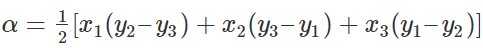It can be represented in the form of the determinant as: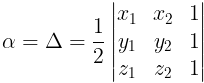System of Linear Equation (Cramer's Rule)

Case 1: System of linear equations in two variables.

Let the system of equations be:

a1x + b1 y + c1 = 0
a2x + b2 y + c2 = 0, where a1/a2 ≠ b1/b2

Solving by cross multiplication, we get,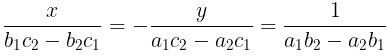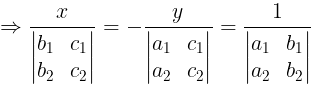Case 2: System of linear equations in three variables.

Let the system of equations be:

a1x + b1y + c1z = d1
a2x + b2y + c2z = d2
a3x + b3y + c3z = d3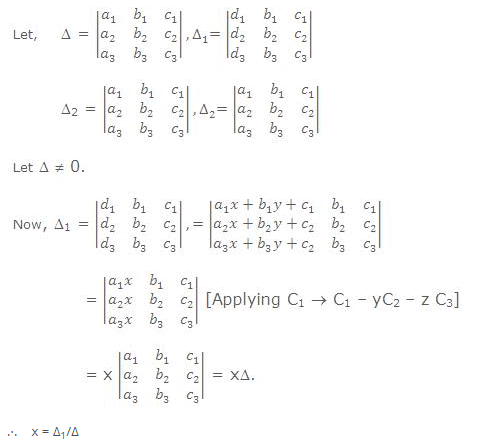Simillarly for y = Δ2/Δ and z = Δ3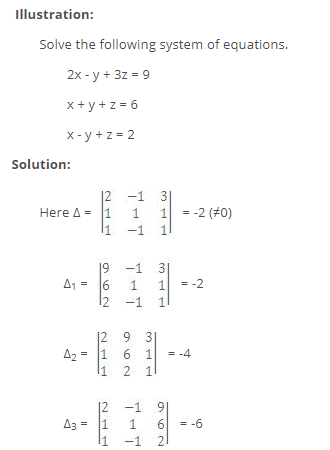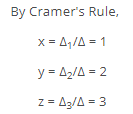Offer running on EduRev: Apply code STAYHOME200 to get INR 200 off on our premium plan EduRev Infinity!

,

,

,

,

,

,

,

,

,

,

,

,

,

,

,

,

,

,

,

,

,

;